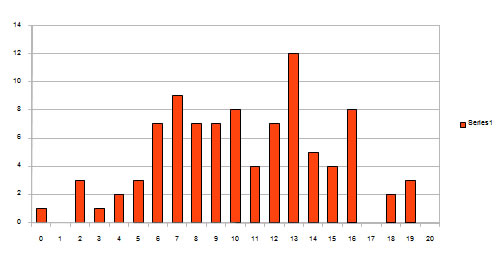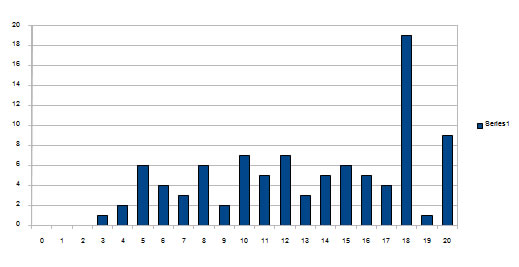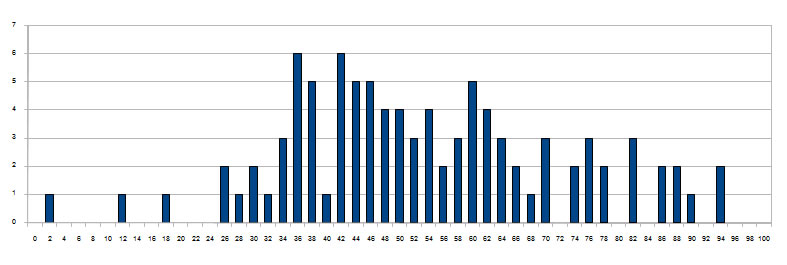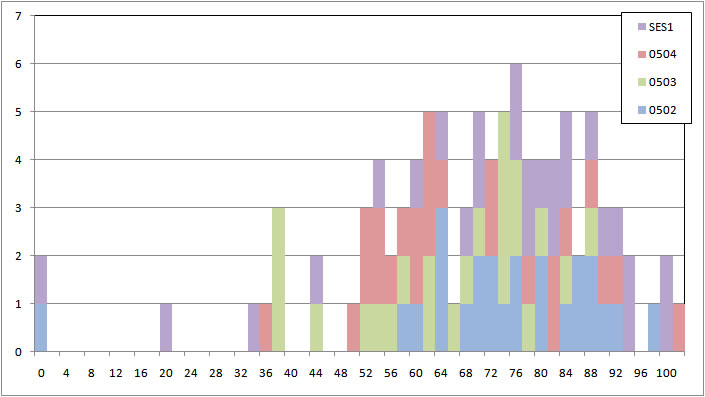Spring 2009Two quantities are reported below. The mean and the standard deviation for each section, and for the class as a whole. So if the mean is 10.3 and the standard deviation is 3.85, then 68 % of the students who took the exam have scores between 10.3-3.85 and 10.3+3.85 (that is between 6.45 and 14.15) and 95 % of the students are between 2.60 (10.3-2*3.85) and 18.0 (10.3+2*3.85)

QUIZZES

Quiz # Section 0502 Section 0503 Section 0504 Section SES1 ALL
Mean Std. Dev Mean Std. Dev Mean Std. Dev Mean Std. Dev Mean Std Dev
1 10.3 3.85 10.2 3.36 9.96 4.13 10.5 5.55 10.23 4.27
2 13.4 4.76 13.2 5.27 12.3 5.21 13.7 4.81 13.14 4.95
3
4
5
6

Quiz 1 DistributionQuiz 2 DistributionEXAMS

Exam# Section 0502 Section 0503 Section 0504 Section SES1 ALL
Mean Std. Dev Mean Std. Dev Mean Std. Dev Mean Std. Dev Mean Std Dev
1 57.7 17.2 46.8 15.9 51.6 4.13 54.5 21.2 52.6 18.6
2 72.8 18.9 64.1 15.0 67.2 16.4 71.1 24.5 68.9 19.2
3

Exam 1 DistributionExam 2 Distibution